Name:    Unit 3 Practice Test

Matching

Match each item with the correct statement below.
 a. mass number d. atomic mass b. atomic mass unit e. isotope c. atomic number

1.

the total number of protons and neutrons in the nucleus of an atom

2.

the number of protons in the nucleus of an element

3.

the average of the masses of the isotopes of an element

4.

atoms with the same number of protons, but different numbers of neutrons in the nucleus of an atom

5.

unit of measurement used to measure an atom’s mass

Match each item with the correct statement below.
 a. proton d. electron b. nucleus e. neutron c. atom

6.

a negatively charged subatomic particle

7.

a subatomic particle with no charge

8.

a positively charged subatomic particle

9.

the central part of an atom, containing protons and neutrons

10.

the smallest particle of an element that retains the properties of that element

Multiple Choice
Identify the choice that best completes the statement or answers the question.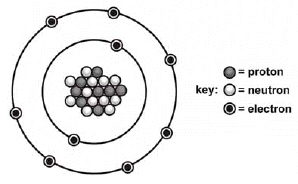11.

What is the mass number of the atom shown above?
 a. 18 b. 20 c. 19 d. 11

12.

Which is the correct symbol for this atom shown above?
 a.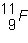b.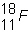c.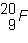d.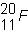13.

Very energetic particles that move in all directions around the nucleus of an atom are
 a. electrons. b. protons. c. neutrons. d. charges.

14.

Balance the following equation: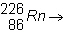____ +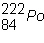a.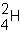b.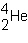c.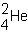d.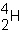15.

The three types of nuclear radiation in order of weakest to strongest are ____.
 a. beta, gamma, alpha c. alpha, beta, gamma b. alpha, gamma, beta d. all of the above

16.

An atom has 12 protons and 13 neutrons. Which is the correct chemical symbol for this atom?
 a.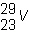b.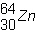c.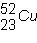d.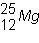17.

Which element has 14 protons?
 a. S b. Si c. Al d. N

18.

Most of the space in an atom is occupied by the
 a. nucleus. b. electrons. c. neutrons. d. protons.

19.

The atomic mass of an element is the average of the masses of its
 a. naturally occurring isotopes. c. artificial isotopes. b. radioactive isotopes. d. two most abundant isotopes.

20.

Which one(s) have a mass of 1 amu and make up the mass number of an atom?
 a. electron and proton c. proton b. electron d. proton and neutron

21.

What single dose of radiation will damage human tissue?
 a. 100 radiators c. 100 rem b. 100 alligators d. 1 x 10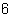rem

22.

What unit measures radiation damage to human tissue?
 a. liter b. rem c. meter d. half-life

23.

The half-life of a radioisotope is the amount of time it takes for
 a. half of a given sample to decay. b. all the sample to decay. c. the age of an artifact to be calculated. d. one half of an atom to decay.

24.

How many electrons are needed to completely fill the second energy level?
 a. 32 b. 2 c. 8 d. 18

25.

Neon-22 contains 12 neutrons. It also contains
 a. 22 electrons. c. 22 protons. b. 12 protons. d. 10 protons.

26.

Which is the smallest subatomic particle, (is has nearly no mass at all)
 a. neutron b. proton c. electron d. atom

27.

Each inner energy level of an atom has a maximum number of ____ it can hold.
 a. electrons b. neutrons c. protons d. quarks

28.

Which of the following is not part of Dalton's atomic theory?
 a. All matter is composed of extremely small particles called atoms. b. Atoms of one element cannot be changed into atoms of another element c. In chemical reactions, atoms combine in whole number ratios.. d. The number of protons in an atom is its atomic number.

29.

What does the 256 in mendelevium-256 represent? (Md=mendelevium)
 a. the neutron number c. the atomic number b. the mass number d. the electron number

30.

During radioactive decay, the nucleus disintegrates into
 a. a lighter and more stable nucleus. c. a heavier and less stable nucleus. b. a heavier and more stable nucleus. d. a lighter and less stable nucleus.

31.

Which best describes Dalton's model of the atom?
 a. a positive mass containing electrons scattered throughout. b. a positive center surrounded by electrons. c. a tiny solid sphere that cannot be split into smaller parts. d. a nuclues containing protons and neutrons surrounded by electrons.

32.

Which of these particles has a mass about equal to a neutron?
 a. proton b. electron c. nucleon d. atom

33.

Which scientist described a positively charged core (“nucleus”) in the middle of a lot of empty space?
 a. Bohr b. Thomson c. Rutherford d. Chadwick

34.

Isotopes are atoms of the same element with different numbers of _________.
 a. charges. b. protons. c. electrons. d. neutrons.

35.

Which scientist described the existence of the neutron?
 a. Thomson b. Chadwick c. Rutherford d. Bohr

36.

What does the 101 in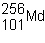represent?
 a. the mass number c. the atomic number b. the number of neutrons d. the nuclide number

37.

Thorium-234 has a half-life of 24 days. If you started with a 100-g sample of thorium-234, how much would remain after 48 days?
 a. 25 g b. 100 g c. 50 g d. 10 g

38.

What is the greatest number of electrons an atom can have in the third energy level?
 a. 0 b. 2 c. 8 d. 18

39.

The type of radiation that can be stopped by a sheet of paper is  ____ radiation.
 a. gamma b. beta c. alpha

40.

Which is the number of neutrons in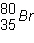?
 a. 80 b. 45 c. 35 d. 115